# JP3867518B2 - Sensorless control system for synchronous motor - Google Patents

## Info

Publication number
JP3867518B2
JP3867518B2 JP2001170425A JP2001170425A JP3867518B2 JP 3867518 B2 JP3867518 B2 JP 3867518B2 JP 2001170425 A JP2001170425 A JP 2001170425A JP 2001170425 A JP2001170425 A JP 2001170425A JP 3867518 B2 JP3867518 B2 JP 3867518B2
Authority
JP
Japan
Prior art keywords
synchronous motor
axis
current
command
inverter
Prior art date
Legal status (The legal status is an assumption and is not a legal conclusion. Google has not performed a legal analysis and makes no representation as to the accuracy of the status listed.)
Active
Application number
JP2001170425A
Other languages
Japanese (ja)
Other versions
JP2002369574A (en
Inventor

Original Assignee

Priority date (The priority date is an assumption and is not a legal conclusion. Google has not performed a legal analysis and makes no representation as to the accuracy of the date listed.)
Filing date
Publication date
Application filed by 株式会社日立製作所 filed Critical 株式会社日立製作所
Priority to JP2001170425A priority Critical patent/JP3867518B2/en
Publication of JP2002369574A publication Critical patent/JP2002369574A/en
Application granted granted Critical
Publication of JP3867518B2 publication Critical patent/JP3867518B2/en
Active legal-status Critical Current
Anticipated expiration legal-status Critical

• 230000001360 synchronised Effects 0.000 title claims description 97
• 230000001276 controlling effects Effects 0.000 claims description 5
• 238000004804 winding Methods 0.000 claims 1
• 238000010586 diagrams Methods 0.000 description 41
• 238000006243 chemical reactions Methods 0.000 description 17
• 230000000875 corresponding Effects 0.000 description 7
• 239000000969 carriers Substances 0.000 description 5
• 239000003990 capacitor Substances 0.000 description 4
• 241001442055 Vipera berus Species 0.000 description 3
• 229910052802 copper Inorganic materials 0.000 description 3
• 239000010949 copper Substances 0.000 description 3
• 238000009499 grossing Methods 0.000 description 3
• 230000000051 modifying Effects 0.000 description 3
• 239000011514 iron Substances 0.000 description 2
• 229910052742 iron Inorganic materials 0.000 description 2
• 229920000729 poly(L-lysine) polymers Polymers 0.000 description 2
• 238000010248 power generation Methods 0.000 description 2
• 239000004065 semiconductors Substances 0.000 description 2
• 230000000593 degrading Effects 0.000 description 1
• 230000001934 delay Effects 0.000 description 1
• 230000003111 delayed Effects 0.000 description 1
• 238000001514 detection method Methods 0.000 description 1
• 230000000694 effects Effects 0.000 description 1
• 238000007562 laser obscuration time method Methods 0.000 description 1
• 230000001429 stepping Effects 0.000 description 1
• 230000001629 suppression Effects 0.000 description 1
• 230000001052 transient Effects 0.000 description 1

## Images

•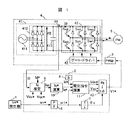•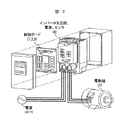•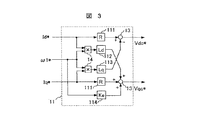•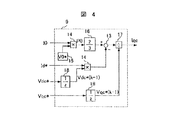••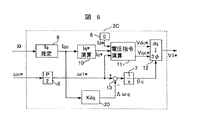•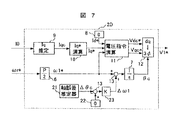••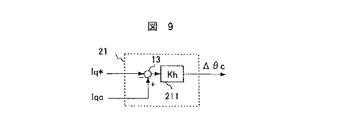•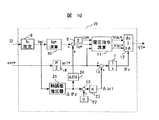•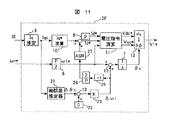•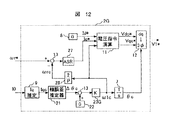•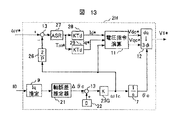•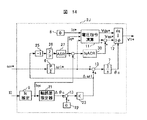••••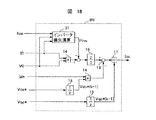•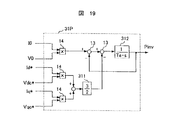•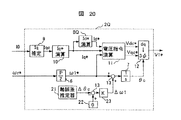•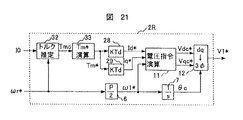••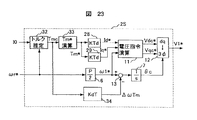•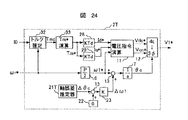•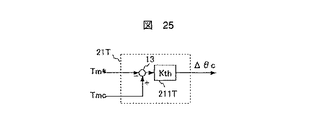•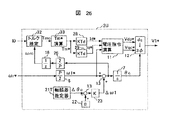•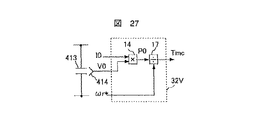•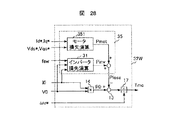•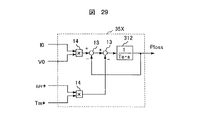•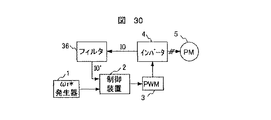•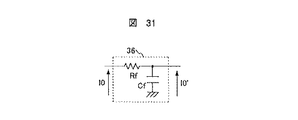•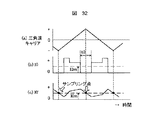•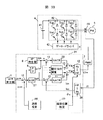•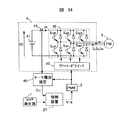•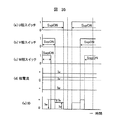## Classifications

• HELECTRICITY
• H02GENERATION; CONVERSION OR DISTRIBUTION OF ELECTRIC POWER
• H02PCONTROL OR REGULATION OF ELECTRIC MOTORS, ELECTRIC GENERATORS OR DYNAMO-ELECTRIC CONVERTERS; CONTROLLING TRANSFORMERS, REACTORS OR CHOKE COILS
• H02P6/00Arrangements for controlling synchronous motors or other dynamo-electric motors using electronic commutation dependent on the rotor position; Electronic commutators therefor
• H02P6/14Electronic commutators
• H02P6/16Circuit arrangements for detecting position
• H02P6/18Circuit arrangements for detecting position without separate position detecting elements
• HELECTRICITY
• H02GENERATION; CONVERSION OR DISTRIBUTION OF ELECTRIC POWER
• H02PCONTROL OR REGULATION OF ELECTRIC MOTORS, ELECTRIC GENERATORS OR DYNAMO-ELECTRIC CONVERTERS; CONTROLLING TRANSFORMERS, REACTORS OR CHOKE COILS
• H02P23/00Arrangements or methods for the control of AC motors characterised by a control method other than vector control
• H02P23/30Direct torque control [DTC] or field acceleration method [FAM]

## Description


BACKGROUND OF THE INVENTION
The present invention relates to a control system for a synchronous motor, and more particularly to a high-accuracy and high-performance synchronous motor drive system having a simplified control configuration without using a sensor for detecting the speed and position of the motor and a motor current sensor.

[Prior art]
FIG. 33 shows a method for controlling the synchronous motor without detecting the magnetic pole position of the synchronous motor or the motor current, and without using a position sensor. In this prior art, a current sensor for detecting the motor current is used instead of the position sensor. In this prior art, based on vector control with a position sensor of a synchronous motor, a magnetic pole position estimator and a speed estimator are provided instead of the position sensor (hereinafter referred to as a vector control type sensorless system). This configuration is exactly the same as the vector control method with a position sensor except for the magnetic pole position detection unit and the speed detection unit.

33, reference numeral 1 is a speed command generator for a rotational speed command ωr *, 2Y is a motor control device, 3 is a PWM generator for converting a voltage command into a PWM (pulse width modulation) pulse, 4 is an inverter, Synchronous motor, 6 is a conversion gain for converting mechanical angular frequency to electrical angular frequency, 8 is an Id * generator for generating d-axis current command Id *, and 11 is for calculating voltage commands Vdc * and Vqc * on the dq axis Voltage command calculator, 12 is a dq inverse converter that converts a value on the dq axis to a three-phase AC value, 13 is an adder that performs addition and subtraction of signals, and 27 is a speed estimated value that matches the speed command. A speed controller 30 for adjusting Iq *, a current controller 30 for correcting the voltage commands Vdc * and Vqc * so that the estimated current values Idc and Iqc coincide with the command values Id * and Iq *, 37 Is the magnetic pole position estimator that estimates the magnetic pole axis of the motor, and 38 is the electric motor 39 is a speed estimator that estimates the rotational speed of the machine, 39 is a dq coordinate converter that converts three-phase alternating current into values on rotational coordinates, 41 is a DC power supply of the inverter, 42 is a main circuit part of the inverter, and 43 is a PWM pulse. A gate driver 44 for turning on and off the semiconductor switching elements Sup to Swn of the inverter main circuit based on the current sensor 44 detects a current of the motor.

33 corresponds to the magnetic pole position sensor, and the speed estimator 38 corresponds to the speed sensor. Further, the speed controller 27 and the current controller 30 are provided in the same manner as the vector control device with a position sensor, and the speed and current are automatically adjusted so as to coincide with each command value. This is, for example, the 2000 Annual Conference of the Institute of Electrical Engineers of Japan, Lecture Collection [III], No. 97, p. 963-966, “Position Sensorless Control of a Permanent Magnet Synchronous Motor by Direct Axis Error Estimation Calculation” It is described in.

The prior art of the control method shown in FIG. 34 using neither a position sensor nor an electric motor current sensor is disclosed in Japanese Patent Laid-Open Nos. 6-153526 and 8-19263. In FIG. 34, reference numeral 40 denotes a motor current estimator that estimates and calculates the motor current from the DC current I0 of the inverter and the PWM pulse waveform, and the other reference numerals are the same as those in FIG.

In FIG. 34, the direct current of the inverter is detected by the current sensor 44 without directly detecting the motor current. The motor current estimator 40 estimates the motor current based on the detected DC current value I0 and the output pulse waveform of the PWM generator 3, and outputs this estimated value I1c to the control device 2Y. In the control device 2Y, vector type sensorless control is performed based on I1c, for example, as in FIG.

Next, the operation of the motor current estimator 40 will be described with reference to FIGS. FIGS. 35A to 35C show the PWM pulse waveform of each phase. When “1”, the positive side switches (Sup, Svp, Swp) are turned on, and when “0”, the negative side is shown. Turn on the switches (Sun, Svn, Swn). Assuming that the motor current is as shown in FIG. 35 (d), the DC current detection value I0 of the inverter has a waveform as shown in FIG. 35 (e). The waveform of FIG. 35 (e) has the following four modes.
Therefore, “Iu” can be detected if a direct current is detected in mode (3), and “Iw” can be detected in mode (2). Iv may be obtained by calculating from Iu and Iw. As described above, the motor current can be reproduced from the switch state of the inverter main circuit and the direct current value, and if the motor current can be estimated, the above-described vector control type sensorless system can be realized.

[Problems to be solved by the invention]
In the case of the vector control type sensorless system shown in FIG. 33, the current sensor 44 is indispensable for the electric motor, and there is a problem of a decrease in reliability due to the use of the sensor 44, or an expensive current for realizing high-precision control. There is a problem in cost, such as the need to use a sensor. In addition, the method of FIG. 32 has a problem of high-frequency oscillation (ringing) of current generated with switching. Since ringing is more likely to occur as the wiring cable of the motor becomes longer, it becomes difficult to detect the current value that should be detected. In addition, under the condition where the rotational frequency of the motor is low, the width of the PWM pulse becomes narrow, and even if the wiring cable is shortened, it becomes susceptible to noise and the detection accuracy is deteriorated.

SUMMARY OF THE INVENTION An object of the present invention is to provide a high-performance synchronous motor drive system that ensures reliability without using a motor current sensor and is hardly affected by noise such as ringing.

[Means for Solving the Problems]
The drive system for a synchronous motor according to the present invention detects a direct current of an inverter that drives the synchronous motor, estimates a torque current component flowing through the motor from the magnitude thereof, and calculates an applied voltage to the motor based on the estimated value. Then, using the estimated value of the torque current, the magnetic pole axis estimation calculation inside the motor is performed.

A drive system for a synchronous motor according to the present invention includes a synchronous motor, an inverter that applies AC to the synchronous motor, a DC power source that supplies power to the inverter, and a means that detects a current supplied to the inverter from the DC power source. A means for giving a rotational speed command to the synchronous motor, a dc axis assuming a magnetic pole axis inside the synchronous motor, and a current command Id * on each axis of the qc axis which is an axis orthogonal to the dc axis, Means for giving a current command Iq *, and means for calculating a voltage command on the dc-qc axis based on the current command Id *, the current command Iq * and the rotation speed command, A control signal is sent to the inverter based on the command, a torque current component inside the synchronous motor is estimated based on the current detection value of the DC power supply, and the current command I is calculated based on the estimated calculation value. Generate q *.

DETAILED DESCRIPTION OF THE INVENTION
Embodiments of the present invention will be described in detail below with reference to the drawings.

Example 1
FIG. 1 shows a configuration diagram of this embodiment. In FIG. 1, reference numeral 1 is a speed command generator for giving a rotational speed command ωr * to the motor, 2 is a control device for calculating the applied voltage of the motor, and 3 is a pulse for driving the inverter 4 based on the voltage command V1 *. Generated PWM (pulse width modulation) generator, 4 is an inverter composed of semiconductor switching elements for driving the motor, 5 is a synchronous motor to be controlled, 6 is a rotational speed command ωr *, and an electrical angular frequency command ω1 * of the motor Conversion gain for conversion (P is the number of poles of the motor), 7 is an integrator for calculating the AC phase θc inside the control device based on the electrical angular frequency command ω1 *, 8 is a magnetic pole axis component (d-axis component) of the motor Id * generator that gives a current command Id * of the motor, 9 is an Iq estimator that estimates and calculates the torque current component of the motor based on the DC current detection value I0 of the inverter, and 10 is the torque current component (q-axis) of the motor. Min) Iq * calculator for calculating the current command Iq * based on the estimated value Iqc, and 11 is a voltage on the dc-qc axis based on the electrical angular frequency command ω1 *, current command Id *, and current command Iq *. A voltage command calculator for calculating commands Vdc * and Vqc *, 12 is a dq inverse converter for converting voltage commands Vdc * and Vqc * on the dc-qc axis into values on a three-phase AC axis, and 41 is an inverter 4 DC power supply unit constituting the main circuit power source of the inverter, 42 is the main circuit unit of the inverter, 43 is a gate driver that generates a gate signal to the main circuit, 44 is a current sensor that detects the DC current of the inverter, 411 is the inverter 4 A three-phase AC power supply 412 for supplying power to a diode bridge rectifying the three-phase AC power supply 413 is a smoothing capacitor for suppressing a pulsating component included in the DC power supply.

The motor drive system of this embodiment shown in FIG. 2 is divided into an AC power source, a control / inverter unit, and an electric motor. As shown in FIG. 2, on the control board in the control / inverter section, the functions shown in the above-mentioned reference numerals 1 to 3 are provided, and these are digital circuits based on a microprocessor. An inverter main circuit unit, a current detection unit, and the like are also mounted in one device.

Next, the operation of this embodiment will be described with reference to FIG. Based on the speed command ωr *, an electric angular frequency command ω1 * of the motor is obtained as an output of the conversion gain 6. The integrator 7 integrates the electrical angular frequency command ω1 * to obtain an AC phase θc inside the controller.

The Iq estimator 9 estimates and calculates the torque current component of the motor based on the detected DC current value I0. The Iq * calculator calculates the current command Iq * based on the estimated torque current component value Iqc of the motor. Calculate. The Id * generator 8 generates a predetermined current command Id *. For example, when the rotor structure of the motor is a non-salient pole type, the current command Id * = 0 is given. The voltage command calculator 11 calculates the voltage command Vdc * and the voltage command Vqc *, which are applied voltages to the synchronous motor 5, based on the electric angular frequency command ω1 * and the current commands Id * and Iq * of the motor using the following equations. To do.

[Expression 1]

The equation (1) is used in normal vector control. For example, “AC servo system theory and design practice”, Hidehiko Sugimoto, General Electronic Publishing, p. 78 (4 .6).

Next, the voltage commands Vdc * and Vqc * obtained by the equation (1) are coordinate-converted to a voltage command value V1 * on the three-phase AC axis using the AC phase θc. The PWM generator 3 converts the voltage command value V1 * into a PWM pulse signal. The gate driver 43 drives the switching element based on this pulse signal, and applies a voltage corresponding to the voltage commands Vdc * and Vqc * to the synchronous motor 5.

Next, the operation of each unit will be described. The voltage command calculator 11 calculates a voltage command based on the formula (1). Expression (1) is represented in a block diagram as shown in FIG. In FIG. 3, reference numeral 13 is an adder (including subtraction), 14 is a multiplier, 111 is a gain corresponding to the resistance value (R) of the motor, 112 is a gain corresponding to the d-axis inductance (Ld), and 113 is q A gain 114 corresponds to the shaft inductance (Lq), and a gain 114 corresponds to the power generation constant (Ke).

As shown in Equation (1) and FIG. 3, the voltage command is calculated using the constants R, Ld, Lq, and Ke of the motor. If these motor constants are accurate, the motor is driven at the rotational speed and current value as instructed. The electrical angular frequency command ω1 * and the current command Id * given to the voltage command calculator 11 can be given regardless of the load state of the motor, but the current command Iq * needs to be given according to the torque required by the motor. Yes, if there is a difference between the current command Iq * and the actual torque load, the magnetic pole axis of the motor will not match the control axis, causing instability and torque shortage. The point of control is whether to create it. In the conventional vector control type sensorless system shown in FIG. 33, the output of the speed controller 27 is the current command Iq *. However, in the case of the vector control type sensorless system, components such as a speed estimator and a speed controller are required, and the control configuration becomes complicated. In this embodiment, the torque current component is estimated from the DC current detection value I0. Then, based on the estimated value Iqc, a current command Iq * is created.

FIG. 4 shows the configuration of the Iq estimator 9. In FIG. 4, reference numerals 13 and 14 are the same as those in FIG. In FIG. 4, reference numeral 15 denotes a V0 * generator that generates a set value V0 of a DC voltage, 16 is a power conversion gain, 17 is a divider, and 18 is a delay device that delays the signal by one sample period.

Next, the operation of FIG. 4 will be described. Regarding the power consumed by the motor, the relationship between the DC power supply unit and the dq coordinate axis is expressed by an equation:

[Expression 2]

become that way. Here, the coefficient 3/2 is a coefficient when relative conversion is used for conversion of dq coordinates. If absolute conversion is used, the coefficient is 1. When formula (2) is transformed,

[Equation 3]

Thus, the torque current component Iq of the motor can be calculated from the DC current detection value I0. However, since the inverter DC voltage V0, the motor voltages Vd and Vq, and the current Id flowing through the motor cannot be directly detected, the set value or command value is used respectively.

[Expression 4]

The torque current component Iq of the electric motor is estimated and calculated. FIG. 4 is a block diagram of Expression (4). As Vdc * and Vqc *, values delayed by the calculation period are used.

Next, a current command Iq * is created using the torque current component estimated value Iqc. Reference numeral 10 in FIG. 1 denotes an Iq * calculator, which calculates a current command Iq * according to the following equation.

[Equation 5]

Equation (5) is a first-order lag filter, but a moving average value or the like may be used in addition to this. When the torque current component estimated value Iqc is directly set as the current command Iq *, the operation is positive and the control system becomes unstable, so that a delay element is added to stabilize. However, since the fundamental wave component (that is, the direct current component) of the torque current component estimated value Iqc and the current command Iq * coincide with each other in a steady state, the magnetic pole axis inside the motor coincides with the control axis, resulting in a stable motor. A drive system can be realized. As described above, according to the present embodiment, it is possible to realize an electric motor drive system capable of stably driving an electric motor with a configuration in which the control configuration is extremely simplified.

(Example 2)
The present embodiment will be described with reference to FIG. FIG. 5 shows the configuration of the control device 2B, and this control device 2B is used in place of the control device 2 of FIG. Reference numerals 6 to 13 in FIG. 5 are the same as those in the first embodiment. Reference numeral 19 denotes an I0 damping gain that adds a correction value ΔωI0 to the electrical angular frequency command ω1 * using the DC current detection value I0 of the inverter. In this embodiment, an I0 damping gain 19 is added to the first embodiment.

Next, the operation of this embodiment will be described. Since the electric power consumption of the electric motor is in the relationship shown in Expression (2), when the electric motor load changes and the electric power consumption increases, the DC current detection value I0 increases if the DC voltage V0 is constant. Therefore, when the DC current detection value I0 increases, a load disturbance occurs, and the motor speed decreases accordingly. In order to cope with load fluctuations without causing the motor to step out, the speed inside the control device may be reduced according to the load. Therefore, if the electrical angular frequency command ω1 * is corrected using the detected DC current value I0, the step-out can be prevented by changing the rotational speed of the electric motor according to the load disturbance.

Since the correction amount ΔωI0 of the electrical angular frequency ω1 * needs to be zero in the steady state, the I0 damping gain 19 may be composed of a differential element or an incomplete differential element. According to the present embodiment, it can be stably driven against load fluctuations.

(Example 3)
The present embodiment will be described with reference to FIG. FIG. 6 shows the configuration of the control device 2C, and this control device 2C is used in place of the control device 2 of the first embodiment. In FIG. 6, reference numerals 6 to 13 are the same as those in the first embodiment, and reference numeral 20 denotes an Iq damping that adds a correction value Δωq to the electrical angular frequency command ω1 * using the estimated torque current value Iqc. • Gain. In this embodiment, an Iq damping gain 20 is added to the first embodiment.

Next, the operation of this embodiment will be described. In the second embodiment, the electric angular frequency command ω1 * is corrected using the DC current detection value I0. However, in the present embodiment, the electric angular frequency command ω1 * is corrected using the torque current estimated value Iqc. The DC current detection value I0 is a physical quantity that changes due to the power consumption of the motor, but is not directly related to the load torque. Therefore, in the region where the motor speed is low, the DC current detection is possible even if the load torque fluctuation is large. The change in value I0 is negligible. Therefore, more accurate compensation of the electrical angular frequency command ω1 * can be realized when the torque current component having a strong correlation with the load torque is used. In this embodiment, the electrical angular frequency command ω1 * is corrected using the estimated torque current component value Iqc to improve the stability against torque disturbance in the low speed range.

Since the correction value Δωq of the electrical angular frequency command ω1 * needs to be zero in the steady state, the Iq damping gain 20 is a differential element or an incomplete differential element as in the case of the I0 damping gain 19. Constitute. According to this embodiment, it is possible to drive stably even with respect to torque load fluctuation in a low speed region.

Example 4
The present embodiment will be described with reference to FIGS. FIG. 7 shows the configuration of the control device 2D, and this control device 2D is used in place of the control device 2 of FIG. In FIG. 7, reference numerals 6 to 13 are the same as those of the same number in the above-described embodiment. Reference numeral 21 denotes an axis error estimator that estimates and calculates an axis error between the magnetic pole axis of the motor and the control axis, 22 a zero generator that gives a zero command to the axis error, and 23 an electrical angular frequency command ω1 * based on the axis error This is the magnetic pole axis estimation gain for calculating the correction amount to

Next, the operation of this embodiment will be described with reference to FIG. In this embodiment, reference numerals 21 to 23 are added to the control device 2 of FIG. The axis error estimator 21 estimates and calculates an error Δθ between the magnetic pole axis in the motor and the magnetic pole axis in the control. As shown in FIG. 8, the error Δθ is defined as an error component of the control axis (defined as dc−qc axis) observed from the dq axis inside the motor. The error estimated value Δθc is calculated according to the following equation.

[Formula 6]
Δθc = Kh (Iqc−Iq *) (Equation 6)
FIG. 9 shows a block that embodies Equation (6), and reference numeral 211 denotes a proportional gain Kh for error calculation. Iq * is the torque current command value that is the basis of the voltage value applied to the motor, and if it matches the current torque current component (estimated value) Iqc, the axis error is zero. Can be considered. If there is a difference between the two, an error Δθ proportional to the difference is generated between the dq axis and the dc−qc axis. Therefore, as shown in Expression (6), the difference between Iq * and Iqc can be calculated, and the estimated error value Δθc can be obtained via the proportional gain Kh.

When the error estimated value Δθc is positive, the control axis dc−qc axis is ahead of the dq axis from the relationship shown in FIG. 8, and therefore the correction amount Δω1 (in this case) so as to reduce the electrical angular frequency command ω1 *. Adds Δω1 <0) and reduces the error Δθ. Conversely, when the error estimated value Δθc is negative, the correction amount Δω1 is added so as to increase the electrical angular frequency command ω1 *. These operations (PLL operation) are realized by the blocks 21 to 23 in FIG. The magnetic pole axis estimation gain 23 is a coefficient for determining the convergence time of the error estimated value Δθc. Basically, it may be a proportional gain, but may be a combination of proportional / integral or differential elements. According to this embodiment, it is possible to compensate for the shaft misalignment and realize higher performance motor control.

(Example 5)
The present embodiment will be described with reference to FIG. FIG. 10 shows the configuration of the control device 2E, and this control device 2E is used instead of the control device 2 of FIG. In FIG. 10, reference numerals 6 to 13 and 21 to 23 are the same as those in the above-described embodiment. Reference numeral 24 denotes a torque controller that calculates the current command Iq * based on the estimated error value Δθc of the magnetic pole axis. The difference between the present embodiment and the fourth embodiment is that a torque controller 24 is added.

Next, the operation of FIG. 10 will be described. In this embodiment, the current command Iq * is determined using the error estimation value Δθc of the magnetic pole axis. Since the phase θc of the AC voltage applied to the motor is mainly given by the integration of the electrical angular frequency command ω1 *, when the load changes suddenly, the error estimated value Δθc changes first. Of course, the electrical angular frequency command ω1 * is corrected via the magnetic pole axis estimation gain 23, but it takes time according to the PLL setting response until it matches the actual speed. Therefore, by quickly determining the current command Iq * from the change in the estimated error value Δθc, highly responsive torque control can be realized.

If the Iq * calculator 10 in FIG. 10 is not used, the estimated error value Δθc is constantly zero, so that an integral element is required inside the torque controller. In this case, the torque controller 24 may be configured based on PI (proportional / integral) control, PID (proportional / integral / differential) control, or the like. On the contrary, when used together with the Iq * calculator, the torque controller may be composed of a proportional element or a differential element. According to the present embodiment, a current command according to the torque change can be obtained quickly.

(Example 6)
The present embodiment will be described with reference to FIG. FIG. 11 shows the configuration of the control device 2F, and this control device 2F is used instead of the control device 2 of FIG. In FIG. 11, reference numerals 6 to 13 and 21 to 23 are the same as those in the above-described embodiment. Reference numeral 25 is an inversion gain that inverts the sign of the signal, 26 is a conversion gain that converts the electrical angular frequency into a mechanical angular frequency, and 27 is a speed controller that controls the speed of the motor to be constant. The difference between the present embodiment and the fifth embodiment is that reference numerals 25 to 27 are added instead of the torque controller 24.

Next, the operation of FIG. 11 will be described. In this embodiment, the current command Iq * is created using the correction amount Δω1 output from the magnetic pole axis estimation gain 23. In this embodiment, in order to quickly converge the speed deviation to zero, the speed controller 27 is provided to create the current command Iq *. The control configuration is simplified by using the correction amount Δω1 of the electrical angular frequency command ω1 * as the speed deviation.

The correction amount Δω1 output by the magnetic pole axis estimation gain 23 is a positive value when the actual speed of the motor is higher than the speed command in the control device, and therefore the sign is inverted via the inversion gain 25. This is equivalent to the input (speed deviation) in the speed controller of the prior art, for example, the speed controller 27 of FIG. Next, the conversion gain 26 divides the correction amount Δω1 by the pole pair number P of the electric motor to convert it into a mechanical angular frequency deviation. Finally, the current command Iq * is calculated using the speed controller 27. The speed controller 27 may use, for example, PI control, PID control, or the like used for speed control in the prior art. Further, when used together with the Iq * calculator, the speed controller may be configured with a proportional or differential element as in the fifth embodiment.

According to the present embodiment, by configuring the speed controller based on the correction amount Δω1 of the electrical angular frequency command ω1 *, it is possible to realize an electric motor drive system with good speed followability.

(Example 7)
This embodiment will be described with reference to FIG. FIG. 12 shows the configuration of the control device 2G, and this control device 2G is used instead of the control device 2 of FIG. In FIG. 12, reference numerals 6-9, 11-13, 21, 22, 26 and 27 are the same as those of the same number in the above-described embodiment. Reference numeral 23G denotes a magnetic pole axis estimation gain composed of elements including integration. The difference between the present embodiment and the sixth embodiment is that the AC phase θc is calculated based on the output of the magnetic pole axis estimation gain 23G instead of having a loop for directly calculating the AC phase θc from the electrical angular frequency command ω1 *. The speed controller 27 calculates the current command Iq * based on the output of the magnetic pole axis estimation gain and the deviation of the rotational speed command ωr *.

Next, the operation of FIG. 12 will be described. In the present embodiment, the magnetic pole axis estimation gain 23G has an integral element, and the AC phase θc is calculated based on the value. As a result, the AC phase θc in the control device changes in accordance with the actual motor speed, so that the motor can be prevented from stepping out.

In control device 2G, torque current component estimated value Iqc is calculated by Iq estimator 9 using DC current detection value I0, and error estimated value Δθc is calculated based on the calculation result. The magnetic pole axis estimation gain 23G outputs the electrical angular frequency ω1c so that the error estimated value Δθc is zero. The magnetic pole axis estimation gain 23G also functions as a speed estimator and performs speed control using this. The estimated speed value ωrc is obtained by passing the conversion gain 26 to the electrical angular frequency ω1c that is the output of the magnetic pole axis estimation gain 23G. A deviation between the estimated speed value ωrc and the rotational speed command ωr * is input to the speed controller 27 to obtain a current command Iq *. In FIG. 12, the speed controller 27 has an integral element.

Here, there is no problem even if the magnetic pole axis estimation gain 23G of this embodiment is used for the above-described embodiment (for example, the embodiment 4 shown in FIG. 7). As described above, according to the present embodiment, it is possible to realize an electric motor drive system that can follow the speed command without depending on the inertia of the electric motor and the load device.

10, 11, and 12, the current command Iq * is obtained using the output of the torque controller 24 or the speed controller 27, but based on these controller outputs, A current command Id * can also be obtained. FIG. 13 is an example in which the current command Id * and the current command Iq * are calculated using the speed controller output for the control device of FIG. In FIG. 13, reference numerals 7, 9, 11 to 13, 21, 22, 23G, 26, and 27 are the same as those having the same numbers in the previous embodiments. Reference numerals 28 and 29 are an Id * data table and an Iq * data table for outputting the current command Id * and the current command Iq * based on the output of the speed controller.

In the case of a salient pole type synchronous motor, the efficiency can be improved by utilizing not only the torque by the permanent magnet but also the reluctance torque utilizing the saliency of the motor. In this case, the current command Id * is not always controlled to zero, but a current command Id * and a current command Iq * that minimize the total current value flowing through the motor are given according to the required torque. In the case of FIG. 13, the output of the speed controller is regarded as a torque command Tm *, and current commands Id * and Iq * necessary for the torque are obtained from the respective data tables. A method for obtaining the current commands Id * and Iq * from the torque command Tm * is described in, for example, FIGS. 2 and 3 of Japanese Patent Laid-Open No. 2000-116198.

(Example 8)
The present embodiment will be described with reference to FIG. FIG. 14 shows a control device 2J, and this control device 2J is used in place of the control device 2 in FIG. In FIG. 14, reference numerals 6 to 9, 11 to 13, 21 to 23, and 25 to 27 are the same as those in the above-described embodiment. Reference numeral 30 denotes a current controller for making the value of the q-axis component estimated value Iqc coincide with the current command Iq *.

Next, the operation of this embodiment will be described. 10 to 13, the current command Iq * can be obtained without using the Iq * calculator 10. Further, since the q-axis component estimated value Iqc itself can be calculated from the DC current detection value I0, it can be automatically controlled so that Iqc coincides with the current command Iq *. In FIG. 14, a current controller 30 is added to the control configuration of FIG. The current controller 30 is used to correct the voltage command Vqc * so that the q-axis component estimated value Iqc matches the current command Iq *. With this configuration, the q-axis component estimated value Iqc can be quickly matched with the current command Iq *, and the control performance is further improved. Note that the current controller for the q-axis component current Iq can also be applied to other embodiments, for example, the embodiments of FIGS.

Example 9
The present embodiment will be described with reference to FIG. FIG. 15 shows the configuration of the axis error estimator 21K, and the estimator 21K is used in place of the axis error estimator 21 in each of the embodiments so far. Reference numerals 13, 14, 17, 18, 111, and 113 in FIG. 15 are the same as those having the same numbers in the previous embodiments.

Next, the operation of this embodiment will be described. In the axial error calculators in the embodiments so far, the error estimated value Δθc is calculated according to the equation (6) (FIG. 9). In this embodiment, the error estimated value Δθc is calculated as follows. The voltage equation of the motor is

[Expression 7]

It is. From the above formula,

[Equation 8]

It is. Further, the relationship between the voltage commands Vdc * and Vqc * and the voltages Vd and Vq on the dq axis of the actual synchronous motor is obtained by using an error Δθ.

[Equation 9]

It becomes. From Equation (8) and Equation (9),

[Expression 10]

Is obtained. Here, when approximating cosΔθ≈1, sinΔθ≈Δθ,

[Expression 11]

Is obtained. In the above equation, ignoring the differential term,

[Expression 12]

Is obtained. When Expression (12) is replaced with Id = Id *, Iq = Iqc and expressed in a block diagram, the configuration of FIG. 15 is obtained, and the error Δθ can be accurately calculated over a wide range of the motor speed. Therefore, the estimation error Δθc is further improved from that in FIG. As a result, a motor drive system with higher accuracy and higher performance can be realized.

(Example 10)
This embodiment will be described with reference to FIG. FIG. 16 shows the configuration of the axis error estimator 21L, and the axis error estimator 21L is used in place of the axis error estimator 21 in the embodiments described so far. Reference numerals 13, 14, 17, 18, 111, and 113 in FIG. 16 are the same as those in the above-described embodiments. Reference numeral 212 in FIG. 16 is a control block for a lead element (differential or incomplete differential element).

Next, the operation of this embodiment will be described. The present embodiment is different from the ninth embodiment only in that the differential term of the equation (11) is not ignored and is configured as shown in FIG. With the configuration of FIG. 16, the principle equation obtained from the voltage equation of the motor is faithfully reproduced including the differential term, so that the axis error calculation can be realized with high accuracy even in a transient state. As a result, a motor drive system with higher accuracy and higher performance can be realized.

(Example 11)
The present embodiment will be described with reference to FIG. FIG. 17 shows the configuration of the Iq estimator 9M, and this Iq estimator 9M is used in place of the Iq estimator 9 in the above embodiments. In FIG. 17, reference numerals 13, 14, 16 to 18 and 413 are the same as those having the same numbers in the above-described embodiment. Reference numeral 414 is a voltage sensor that detects the voltage of the smoothing capacitor 413.

The difference between the present embodiment and FIG. 4 is that a voltage sensor 414 for detecting the DC voltage of the inverter is provided, and the torque current estimation calculation is performed using the detected value V0. According to the present embodiment, since Iqc can be calculated using the detected value of the DC voltage, robust control can be performed against fluctuations in the DC voltage.

(Example 12)
The present embodiment will be described with reference to FIG. FIG. 18 shows the configuration of the Iq estimator 9N, and this estimator 9N is used in place of the Iq estimator 9 in the previous embodiments. In FIG. 18, reference numerals 13, 14, and 16 to 18 are the same as those in the above-described embodiment. Reference numeral 31 denotes an inverter loss calculator for calculating a loss consumed by the inverter. The difference between this embodiment and FIGS. 9 and 17 is that an inverter loss calculator 31 is added.

In the present embodiment, the q-axis component estimated value Iqc is calculated in consideration of the loss consumed by the inverter. Expression (13) shows a relational expression of power including the inverter loss Pinv.

[Formula 13]

In the above equation, fsw is an average switching frequency of the inverter, Kv is a coefficient corresponding to the switching loss generated by switching, and Ki is a loss coefficient generated depending on the magnitude of the current flowing through the inverter. When the q-axis component estimated value Iqc is calculated based on Expression (13), the following expression is obtained.

[Expression 14]

When Expression (14) is represented by an actual block diagram, the configuration shown in FIG. 18 is obtained. The coefficients Kv and Ki for calculating Pinv in equations (13) and (14) are experimentally determined and set in advance.

As described above, by using the Iq estimator 9N shown in FIG. 18, the q-axis component estimated value Iqc can be estimated with higher accuracy in consideration of the loss generated in the inverter.
(Example 13)
The present embodiment will be described with reference to FIG. FIG. 19 shows the configuration of the inverter loss calculator 31P, and this calculator 31P is used in place of the inverter loss calculator 31 in FIG. Reference numerals 13 and 14 in FIG. 19 are the same as those in the above-described embodiment. Reference numeral 311 denotes a power conversion gain, and 312 denotes a loss estimator using an integrator (time constant Te). In this embodiment, the inverter loss is estimated online, and the q-axis component estimated value Iqc is calculated.

Since the difference between the left side (DC input power) in equation (13) and the first term on the right side (power consumption of the motor) is the power consumed by the inverter, the difference between the input and output power is calculated, As the loss Pinv, the q-axis component estimated value Iqc is corrected. In FIG. 19, the DC power consumption calculation and the motor power consumption calculation are performed, and the difference between them is integrated to estimate the inverter loss Pinv.

As described above, by using the inverter loss calculator 31P having the configuration shown in FIG. 19, the loss generated in the inverter can be calculated online, and the q-axis component estimated value Iqc can be accurately calculated.

(Example 14)
The present embodiment will be described with reference to FIG. FIG. 20 shows a configuration of the control device 2Q, and this control device 2Q is used instead of the control device 2 in FIG. In FIG. 20, reference numerals 6, 7, 9 to 13, 21 to 23 are the same as those of the same number in the above-described embodiment. Reference numeral 8Q denotes an Id * generator that determines the value of the current command Id * based on the current command Iq *.

Next, the operation of this embodiment will be described. Some permanent magnet type motors generate electric motor torque by combining the torque of a permanent magnet and the reluctance torque due to the saliency (reverse saliency) of the motor. In the case of this type of motor, there is a maximum torque point of the motor in a range in which the motor current Id flows negatively, and it is not a good idea in terms of efficiency to control to Id = 0. That is, when the electric motor is always driven with the minimum current, that is, the maximum efficiency, the electric motor is always driven with the maximum torque. Conditions for obtaining the maximum torque are described in, for example, “Comparison of PM motor control method and rotor structure characteristics”, IEEJ Transactions D, 1994, Vol. 114, No. 6, pp. 662-667. According to equation (5) in the literature:

[Expression 15]

When the torque current component Iq is determined, the motor current Id for obtaining the maximum torque is determined.

In this embodiment, the Id * generator 8Q can calculate the motor current Id by the equation (15) using the current command Iq * and drive the motor with the maximum torque, that is, the maximum efficiency. Although the q-axis component estimated value Iqc can be used in the calculation of the equation (15) instead of the current command Iq *, the fluctuation of the q-axis component estimated value Iqc at the time of transition is so severe that the entire control system is unstable. There is a risk of becoming. Moreover, since it is sufficient in practice to apply for the maximum efficiency in a steady state, there is no problem even if the current command Iq * output from the Iq * calculator is used. As described above, according to this embodiment, the motor efficiency can be maximized.

(Example 15)
A present Example is described using FIG. 21, FIG. FIG. 21 shows the configuration of the control device 2R, and this control device 2R is used in place of the control device 2 of FIG. In FIG. 21, reference numerals 6, 7, 11, 12, 28, and 29 are the same as those of the same number in the above-described embodiment. Reference numeral 32 denotes a torque estimator that estimates and calculates the torque generated by the motor based on the detected DC current value I0, and reference numeral 33 denotes a Tm * calculator that calculates the torque command Tm * based on the estimated torque value Tmc.

In this embodiment, the generated torque of the electric motor is directly estimated and calculated. The relationship between the generated torque of the motor and the output Pm is

[Expression 16]
Pm = ωrTm (Expression 16)
Where Tm: generated torque of the motor, ωr: rotational speed of the motor
It becomes. Therefore, since the power consumption on the DC side is equal to Equation (16),

[Expression 17]

Thus, the motor torque can be estimated and calculated directly. Expression (17) is represented in a block diagram as shown in FIG.

In FIG. 21, the torque estimator 32 estimates and calculates the motor torque directly from the detected DC current value I0, and then calculates the torque command Tm * with the Tm * calculator. In the calculation of the torque command Tm *, a delay element (for example, Expression (5)) is used as in the first embodiment. If the torque command Tm * is obtained, the current commands Id * and Iq * necessary for the torque command Tm * are created using the data tables 28 and 29. The data tables 28 and 29 may be the same as those described in the seventh embodiment (FIG. 13).

According to the present embodiment, it is possible to estimate the generated torque of the motor using a torque estimator, and as a result, it is possible to realize the motor efficiency maximization control with a simple control configuration, which is simple and versatile. High control is possible.

(Example 16)
The present embodiment will be described with reference to FIG. FIG. 23 shows a configuration of the control device 2S, and this control device 2S is used in place of the control device 2 in FIG. In FIG. 23, reference numerals 6, 7, 11, 12, 28, 29, 32, and 33 are the same as those in the above-described embodiment. Reference numeral 34 denotes a torque damping gain that adds a correction value Δωq to the electrical angular frequency command ω1 * using the estimated torque value Tmc. In this embodiment, a torque damping gain 34 is added to the embodiment of FIG.

In the torque estimator 32, since the actual motor torque can be observed instantaneously, the load fluctuation of the motor appears as a change in the estimated torque value Tmc. Therefore, the electrical angular frequency command ω1 * is corrected using the estimated torque value Tmc. Then, the step-out can be prevented by changing the rotation speed of the electric motor according to the load disturbance. Since the correction amount ΔωTm of the electrical angular frequency command ω1 * needs to be zero in the steady state, the torque damping gain 34 may be constituted by a differential element or an incomplete differential element.

As described above, according to the present embodiment, it is possible to drive stably even with respect to load fluctuation.

(Example 17)
A present Example is described using FIG. 24 and FIG. FIG. 24 shows the configuration of the control device 2T, and this control device 2T is used in place of the control device 2 of FIG. In FIG. 24, reference numerals 6, 7, 11 to 13, 22, 23, 28, 29, 32, and 33 are the same as those in the above-described embodiment. Reference numeral 21T denotes an axis error estimator that estimates and calculates an axis error between the magnetic pole axis of the motor and the control axis.

Next, the operation of FIG. 24 will be described. This embodiment is obtained by adding reference numerals 21T, 22, and 23 to the control device 2R of FIG. The shaft error estimator 21T calculates the error estimated value Δθc by the following formula based on the torque command Tm * and the torque estimated value Tmc.

[Formula 18]
Δθc = Kth (Tmc−Tm *) (Equation 18)
FIG. 25 shows a block that embodies Equation (18). In FIG. 25, reference numeral 211T denotes a proportional gain Kth for calculating an axis error. If the torque command Tm * matches the estimated torque value Tmc at that time, the error Δθ can be regarded as zero. If there is a difference between the two, an error Δθ proportional to the difference is generated between the dq axis and the dc−qc axis. Therefore, as shown in the equation (18), the error Δθ can be estimated by calculating the difference between the torque command Tm * and the estimated torque value Tmc and via the proportional gain Kth. Once the estimated error value Δθc is obtained, the estimated error value Δθc can be controlled to zero by correcting the AC phase θc via the magnetic pole axis estimation gain 23 as in the previous embodiments.

(Example 18)
This embodiment will be described with reference to FIG. FIG. 26 shows a configuration of the control device 2U, and this control device 2U is used instead of the control device 2 in FIG. In FIG. 26, reference numerals 6, 7, 11 to 13, 18, 21T, 22, 23, 26, 28, 29, 32, and 33 are the same as those in the above-described embodiment. This embodiment is different from the embodiment of FIG. 24 in that the rotation speed used for the torque estimation calculation is not the rotation speed command ωr * but the rotation speed estimated value ωrc.

As shown in FIG. 26, the estimated rotational speed value ωrc is obtained by converting the electrical angular frequency obtained by adding the correction amount Δω1 to the electrical angular frequency command ω1 * into the mechanical angular frequency via the conversion gain 26. As shown in Equation (16), the relationship between the torque of the motor and the electric power can be obtained in principle by using the actual rotational speed, so that an accurate value can be obtained from the rotational speed command. Therefore, it is possible to estimate the torque with higher accuracy. As described above, according to this embodiment, the torque can be estimated with higher accuracy.

Example 19
This embodiment will be described with reference to FIG. FIG. 27 shows the configuration of the torque estimator 32V, and this torque estimator 32V is used in place of the torque estimator 32 in the embodiments so far. In FIG. 27, reference numerals 14, 17, 413, and 414 are the same as those in the above-described embodiment. In the torque estimator of this embodiment, a voltage sensor 414 for detecting the DC voltage of the inverter is provided, and the torque estimated value Tmc is calculated using the DC voltage detected value V0. According to the present embodiment, since the estimated torque value Tmc can be calculated using the detected value of the DC voltage, robust control can be performed with respect to fluctuations in the power supply voltage and fluctuations in the DC voltage caused by fluctuations in the motor load.

(Example 20)
This embodiment will be described with reference to FIG. FIG. 28 shows a configuration of the torque estimator 32W, and this torque estimator 32W is used in place of the torque estimator 32 or the torque estimator 32V in the embodiments so far. In FIG. 28, reference numerals 13, 14, 17, and 31 are the same as those in the above-described embodiment. Reference numeral 35 denotes a total loss calculator that calculates all loss Ploss other than the torque output of the motor, and 351 denotes a motor loss calculator that calculates copper loss and iron loss of the motor.

The difference between this embodiment and FIG. 22 or FIG. 27 is that a total loss calculator 35 including an inverter loss calculator 31 and a motor loss calculator 351 is added. In the present embodiment, as in the embodiment of FIG. 18, a total loss calculator 35 for calculating all of the loss is provided to calculate the estimated torque value Tmc. Since the inverter loss calculator 31 in the total loss calculator is the same as that in the above-described embodiment, the description thereof is omitted. The motor loss calculator 351 calculates the motor loss Pmot according to the following equation.

[Equation 19]
Ploss = Kr (Id * 2 + Iq * 2 ) + Km (Vdc * 2 + Vqc * 2 ) (Equation 19)
In the above equation, Kr is the loss due to the copper loss of the motor, and Krm is the loss due to the copper loss of the iron loss. The total loss Ploss is

[Expression 20]
Ploss = Pinv + Pmot (Equation 20)
It becomes. When the torque is estimated in consideration of the loss in the above equation, the configuration shown in FIG. 28 is obtained. As described above, by using the torque estimator having the configuration shown in FIG. 28, it is possible to consider the loss generated in the inverter and the electric motor, and the torque estimated value Tmc can be calculated with higher accuracy.

(Example 21)
This embodiment will be described with reference to FIG. FIG. 29 shows the configuration of the total loss calculator 35X, and this calculator 35X is used in place of the total loss calculator 35 in FIG. In FIG. 29, reference numerals 13, 14, and 312 estimate on-line all losses other than the torque output that are the same as those of the same number in the above-described embodiment, and improve the accuracy of the torque estimation calculation. The relationship between DC input power P0, total loss Ploss, and torque Tm is

[Expression 21]
P0 = ωr · Tm + Ploss (Expression 21)
Therefore, the loss component can be obtained online by calculating the difference between the torque output Pm (= ωr · Tm) and the input power P0 and estimating Ploss. FIG. 29 shows the implementation of this operation. In FIG. 29, the power consumption calculation on the DC side and the torque output calculation of the motor are performed, and the difference between them is integrated to estimate the total loss Ploss.

As described above, by using the inverter loss calculator of this embodiment, it is possible to calculate a loss other than the torque output online, and the estimated torque value Tmc can be estimated more accurately.

(Example 22)
A present Example is described using FIG. 30, FIG. FIG. 30 shows the configuration of the synchronous motor drive system of the present embodiment. In the present embodiment, a filter 36 is added to the detected DC current value I0 in the previous embodiments. The filter 36 deletes harmonic components contained in the detected DC current value I0. The filter 36 is, for example, a first-order lag filter including a capacitor Cf and a resistor Rf shown in FIG.

The actual DC current detection value I0 is, for example, a waveform with a pulsating component as shown in FIG. Since the value necessary for realizing the embodiments so far is the average value of the direct current (I0m in FIG. 32B), it is difficult to directly use the detected direct current value I0 for the calculation. Thus, for example, it is conceivable to sample the direct current in a time sufficiently shorter than the period of the triangular wave carrier and obtain the average value thereof, but this is not practical because it requires high-speed arithmetic processing. Therefore, the filter shown in FIG. 31 is inserted to eliminate the pulsation component accompanying the switching of the inverter and detect the average value of the direct current. Note that if the time constant Tf (= Cf · Rf) of the filter is set longer than the average switching period of the inverter (period of the triangular wave carrier), the main pulsation component can be removed. In addition, the filter of a present Example can be implemented with respect to all the Examples demonstrated so far.

(Example 23)
This embodiment will be described with reference to FIG. The present embodiment is a DC current detection method that minimizes the filter time constant and is not affected by the pulsating component. FIG. 32 shows operation waveforms of the twenty-second embodiment. FIG. 32A is a triangular wave carrier used when creating a PWM pulse waveform, FIG. 32B is a DC current detection value I 0, and FIG. 32C is a DC current detection value after passing through the filter 36. This is the waveform of I0 (I0 '). Since the filter time constant is set to the minimum necessary, harmonic ripple components remain in I0 ′.

The detected value I0 of the direct current shown in FIG. 32B is a waveform synchronized with the triangular wave carrier, and is always zero at the peak of the triangular wave carrier (positive side, negative side, both peaks). When the detected value I0 of the DC current is zero, that is, all the switching elements of the inverter are on (Sup, Svp, Swp in FIG. 1 are all on), or all the lower sides are on (Sun, FIG. 1). Svn and Swn are all on). Under this condition, the DC power supply and the inverter are disconnected.

During the period when the DC current detection value I0 is zero (period t0 in FIG. 32), the filtered current I0 ′ changes gently and always crosses the average value I0m. Therefore, if the I0 ′ is sampled at the timing of the t0 period and the value is used for the control, the average value of the DC current detection value I0 necessary for the control can be read, so the filtered current I0 There is no problem even if some ripples are included in ′. Therefore, according to the present embodiment, a high-performance motor drive system can be achieved without setting the DC current detection filter time constant to a minimum and degrading the control response.

【The invention's effect】
According to the motor drive system of the present invention, high-performance and high-precision motor drive can be realized with a simple control configuration without using a position / speed sensor and a motor current sensor for controlling the motor. As a result, the reliability and stability of the motor drive system are improved.
[Brief description of the drawings]
FIG. 1 is a configuration diagram of Embodiment 1. FIG.
FIG. 2 is a schematic structural diagram of an apparatus in which the motor drive system according to the first embodiment is mounted.
FIG. 3 is a configuration diagram of a voltage command calculator according to the first embodiment.
4 is a configuration diagram of an Iq estimator according to Embodiment 1. FIG.
5 is a configuration diagram of Example 2. FIG.
6 is a configuration diagram of Embodiment 3. FIG.
7 is a configuration diagram of Embodiment 4. FIG.
FIG. 8 is a vector diagram showing a relationship between a dq axis in the electric motor, a dc-qc axis in the control device, and an error Δθ.
FIG. 9 is a configuration diagram of an axis error estimator according to the fourth embodiment.
10 is a configuration diagram of Embodiment 5. FIG.
11 is a configuration diagram of Embodiment 6. FIG.
12 is a configuration diagram of Embodiment 7. FIG.
FIG. 13 is another configuration diagram of the seventh embodiment.
14 is a configuration diagram of Embodiment 8. FIG.
15 is a configuration diagram of Embodiment 9. FIG.
16 is a configuration diagram of Example 10. FIG.
17 is a configuration diagram of Example 11. FIG.
18 is a configuration diagram of Example 12. FIG.
19 is a configuration diagram of Example 13. FIG.
20 is a configuration diagram of Example 14. FIG.
FIG. 21 is a configuration diagram of Example 15.
22 is a configuration diagram of a torque estimator according to Embodiment 15. FIG.
23 is a configuration diagram of Example 16. FIG.
24 is a configuration diagram of Example 17. FIG.
25 is a configuration diagram of an axis error estimator according to Embodiment 17. FIG.
26 is a configuration diagram of Example 18. FIG.
27 is a configuration diagram of Example 19. FIG.
28 is a configuration diagram of Example 20. FIG.
29 is a block diagram of Example 21. FIG.
30 is a configuration diagram of Example 22. FIG.
31 is a configuration diagram of a filter of Example 23. FIG.
32 is a diagram showing operation waveforms in Example 23. FIG.
FIG. 33 is a block diagram of a conventional synchronous motor vector control system without position / speed sensors.
FIG. 34 is a block diagram of a synchronous motor drive system that does not use a conventional motor current sensor.
FIG. 35 is a diagram showing the operation of a synchronous motor drive system that does not use a motor current sensor of the prior art.
[Explanation of symbols]
DESCRIPTION OF SYMBOLS 1 ... Speed command generator, 2, 2B, 2C, 2D, 2E, 2F, 2G, 2Q, 2R, 2S, 2T, 2U, 2Y ... Control device, 3 ... PWM (pulse width modulation) generator, 4 ... Inverter 5 ... synchronous motor, 6 ... conversion gain (P is the number of poles of the motor), 7 ... integrator, 8, 8Q ... Id * generator, 9, 9M, 9N ... Iq estimator, 10 ... Iq * calculator, DESCRIPTION OF SYMBOLS 11 ... Voltage command calculator, 12 ... dq inverse converter, 13 ... Adder, 14 ... Multiplier, 15 ... V0 * generator, 16 ... Power conversion gain, 17 ... Divider, 18 ... Delayer, 19 ... I0 damping gain, 20 ... Iq damping gain, 21, 21K, 21L, 21T ... Axis error estimator, 22 ... Zero generator, 23,23G ... Magnetic pole axis estimation gain, 24 ... Torque controller, 25 ... Inverting gain , 26 ... conversion gain, 27 ... speed controller, 28 ... I * Data table, 29 ... Iq * data table, 30 ... Current controller, 31, 31P ... Inverter loss calculator, 32,32V, 32W ... Torque estimator, 33 ... Tm * Calculator, 34 ... Torque damping gain 35, 35X ... Total loss calculator, 36 ... Filter, 37 ... Magnetic pole position estimator, 38 ... Speed estimator, 39 ... dq coordinate converter, 40 ... Motor current estimator, 41 ... DC power supply, 42 ... Inverter Main circuit unit 43 ... Gate driver 44 ... Current sensor 111 ... Gain corresponding to electric motor resistance (R) 112 ... Gain corresponding to d-axis inductance (Ld) 113 ... q-axis inductance (Lq) , Gain corresponding to power generation constant (Ke), 211... Proportional gain Kh, 211... Advanced element control block, 211 T. Gain Kth, 311 ... power conversion gain, 312 ... loss estimator 351 ... motor loss calculator, 411 ... three-phase AC power supply, 412 ... diode bridge, 413 ... smoothing capacitor, 414 ... voltage sensor.

## Claims (14)

1. A synchronous motor, an inverter for applying alternating current to the synchronous motor, a DC power supply for supplying power to the inverter, means for detecting a current supplied from the DC power supply to the inverter, and a rotational speed command to the synchronous motor Means for giving, a dc axis assuming a magnetic pole axis inside the synchronous motor, and means for giving a current command Id * and a current command Iq * on the respective axes of the qc axis which is an axis orthogonal to the dc axis; Means for calculating a voltage command on the dc-qc axis based on the current command Id *, the current command Iq * and the rotation speed command, and sending a control signal to the inverter based on the voltage command. In the synchronous motor drive system for controlling the synchronous motor,
A synchronous motor that estimates and calculates a torque current component inside the synchronous motor based on a current detection value of the DC power supply, and generates a current command Iq * of the qc-axis component based on the estimated calculation value Driving system.
2. A synchronous motor, an inverter for applying alternating current to the synchronous motor, a DC power supply for supplying power to the inverter, means for detecting a current supplied from the DC power supply to the inverter, and a rotational speed command to the synchronous motor A current command Id on each of a dc axis assuming a magnetic pole axis in the synchronous motor and a qc axis which is an axis orthogonal to the dc axis. * And current command Iq * And the current command Id * And current command Iq * And a means for calculating a voltage command on the dc-qc axis based on the rotational speed command, and a synchronous motor drive for controlling the synchronous motor by sending a control signal to the inverter based on the voltage command In the system,
A torque current component inside the synchronous motor is estimated based on the detected current value of the DC power source, and the qc axis component current command Iq is calculated based on a value obtained by adding a delay element to the estimated calculated value. * Synchronous motor drive system characterized by generating
3. A synchronous motor, an inverter for applying an alternating current to the synchronous motor, a DC power supply for supplying power to the inverter, a means for detecting a current supplied from the DC power supply to the inverter, and a rotational speed for the synchronous motor A means for giving a command, a means for calculating an AC phase of the synchronous motor based on the rotational speed command, and a means for calculating a voltage command to be applied to the synchronous motor, and the inverter based on the voltage command In a synchronous motor drive system for sending a control signal to drive the synchronous motor,
A synchronous motor drive system characterized in that a torque current component inside the synchronous motor is estimated based on a detected current value of the DC power source, and the rotational speed command is compensated based on the estimated calculated value.
4. According to claim 1 or 2 either synchronous motor driving system, the current detection value of the DC power source, or using an estimated value of the torque current component, the synchronous motor inside the pole axis (d-axis), the dc A synchronous motor drive system characterized in that an error Δθ with a shaft is calculated, and the rotational speed command is compensated based on the error Δθ.
5. A synchronous motor, an inverter for applying alternating current to the synchronous motor, a DC power supply for supplying power to the inverter, means for detecting a current supplied from the DC power supply to the inverter, and a rotational speed command to the synchronous motor Means for giving, a dc axis assuming a magnetic pole axis (d axis) inside the synchronous motor, and means for giving a current command Id * and a current command Iq * on the qc axis which is an axis orthogonal to the dc axis; Means for calculating a voltage command on the dc-qc axis based on the current command Id *, current command Iq * and the rotational speed command, and sending a control signal to the inverter based on the voltage command. In the synchronous motor drive system for controlling the synchronous motor,
Based on the detected current value of the DC power supply, means for estimating a torque current component that is a component orthogonal to the magnetic pole axis in the synchronous motor, and using the estimated value of the torque current component, the d axis, A synchronous motor drive system comprising: means for calculating an error Δθ with respect to the dc axis; and means for generating the current command Iq * based on the error Δθ.
6. A synchronous motor, an inverter for applying alternating current to the synchronous motor, a DC power supply for supplying power to the inverter, means for detecting a current supplied from the DC power supply to the inverter, and a rotational speed command to the synchronous motor A means for calculating an AC phase of the synchronous motor based on the rotational speed command, a dc axis assuming a magnetic pole axis (d axis) inside the synchronous motor, and an axis orthogonal to the dc axis A means for giving a current command Id * and a current command Iq * on the qc axis, and a means for calculating a voltage command on the dc-qc axis based on the current command and the rotation speed command. In the synchronous motor drive system that controls the synchronous motor by sending a control signal to the inverter based on the voltage command,
Based on the detected current value of the DC power supply, means for estimating a torque current component that is a component orthogonal to the magnetic pole axis in the synchronous motor, and using the estimated value of the torque current component, the d axis, Means for calculating an error Δθ with respect to the dc axis, correcting the AC phase based on the error Δθ, and generating a current command Iq * based on the correction amount for correcting the AC phase; Synchronous motor drive system characterized by
7. A synchronous motor, an inverter for applying alternating current to the synchronous motor, a DC power supply for supplying power to the inverter, means for detecting a current supplied from the DC power supply to the inverter, and a rotational speed command to the synchronous motor Means for giving, a dc axis assuming a magnetic pole axis (d axis) inside the synchronous motor, and means for giving a current command Id * and a current command Iq * on the qc axis which is an axis orthogonal to the dc axis; Means for calculating a voltage command on the dc-qc axis based on the current command and the rotation speed command, and based on the voltage command, sends a control signal to the inverter to control the synchronous motor In the synchronous motor drive system,
Based on the current detection value of the DC power supply, the d-axis is calculated using means for estimating a torque current component Iq that is a component orthogonal to the magnetic pole axis in the synchronous motor, and the estimated value Iqc of the torque current component. And an error Δθ with respect to the dc axis, a means for estimating the rotational speed of the synchronous motor based on the error Δθ, and an AC phase of the synchronous motor based on the estimated value of the rotational speed And a means for generating a current command Iq * based on a deviation between the rotation speed command and the rotation speed estimated value.
8.   The synchronous motor drive system according to any one of claims 5 to 7, further comprising means for correcting the voltage command based on a deviation between the current command Iq * and the estimated value Iqc of the torque current component. Synchronous motor drive system.
9. 9. The synchronous motor drive system according to claim 4, wherein the error Δθc is calculated by the following equation (Equation 1).
Where Vdc * is the voltage command on the dc axis, Vqc * is the voltage command on the qc axis, ω1 is the electrical angular frequency of the motor, R is the winding resistance of the motor, Lq is the q-axis inductance of the motor, Iqc is an estimated value of torque current.
10. 9. The synchronous motor drive system according to claim 4, wherein the error Δθc is calculated by the following equation (Equation 2).
However, in the above formula, p is a differential operator.
11.   The synchronous motor drive system according to any one of claims 1 and 3 to 10, further comprising means for detecting a voltage of the DC power supply, and using the detected voltage value, a torque inside the synchronous motor. A synchronous motor drive system characterized by calculating a current component torque current component.
12.   12. The synchronous motor drive system according to claim 1, wherein a torque current component inside the synchronous motor is calculated by subtracting a loss consumed by the inverter. Electric motor drive system.
13.   In the synchronous motor drive system according to claim 12, from the difference between the means for calculating the DC input power of the inverter, the means for calculating the power consumption of the synchronous motor, and the DC input power and the power consumption of the synchronous motor, Means for calculating a loss of the inverter, and a torque current component inside the synchronous motor is calculated using the estimated value of the loss of the inverter.
14.   In the synchronous motor drive system according to any one of claims 1 or 3 to 13, using any one of the DC current detection value, the current command Iq *, and the estimated value Iqc of the torque current component, A synchronous motor drive system which calculates a voltage command Id * on the dc axis.
JP2001170425A 2001-06-06 2001-06-06 Sensorless control system for synchronous motor Active JP3867518B2 (en)

## Priority Applications (1)

Application Number Priority Date Filing Date Title
JP2001170425A JP3867518B2 (en) 2001-06-06 2001-06-06 Sensorless control system for synchronous motor

## Applications Claiming Priority (2)

Application Number Priority Date Filing Date Title
JP2001170425A JP3867518B2 (en) 2001-06-06 2001-06-06 Sensorless control system for synchronous motor
US10/053,694 US6690137B2 (en) 2001-06-06 2002-01-24 Sensorless control system for synchronous motor

## Publications (2)

Publication Number Publication Date
JP2002369574A JP2002369574A (en) 2002-12-20
JP3867518B2 true JP3867518B2 (en) 2007-01-10

# Family

## Family Applications (1)

Application Number Title Priority Date Filing Date
JP2001170425A Active JP3867518B2 (en) 2001-06-06 2001-06-06 Sensorless control system for synchronous motor

## Country Status (2)

US (1) US6690137B2 (en)
JP (1) JP3867518B2 (en)

## Cited By (1)

* Cited by examiner, † Cited by third party
Publication number Priority date Publication date Assignee Title
US8441220B2 (en) 2010-09-21 2013-05-14 Denso Corporation Control device for electric rotating machine

## Families Citing this family (70)

* Cited by examiner, † Cited by third party
Publication number Priority date Publication date Assignee Title
JP4575547B2 (en) * 2000-04-18 2010-11-04 トヨタ自動車株式会社 Motor control device
DE10160612A1 (en) * 2001-12-11 2003-06-26 Siemens Ag traction drive
US6873126B2 (en) * 2002-07-01 2005-03-29 Matsushita Electric Industrial Co., Ltd. Motor drive method and motor driver
JP2004282969A (en) 2003-03-19 2004-10-07 Hitachi Home & Life Solutions Inc Control apparatus and method for ac motor
JP3783695B2 (en) * 2003-03-20 2006-06-07 日産自動車株式会社 Motor control device
JP3771544B2 (en) * 2003-03-24 2006-04-26 株式会社日立産機システム Method and apparatus for controlling permanent magnet type synchronous motor
DE10333414A1 (en) * 2003-07-15 2005-02-10 Sauter Feinmechanik Gmbh Method for operating a motorized positioning device and associated positioning device
KR100634588B1 (en) * 2003-12-30 2006-10-13 현대자동차주식회사 Control system and method for permanent magnet synchronous motor
TWM257255U (en) * 2004-03-01 2005-02-21 Rexon Ind Corp Ltd Emery disk/emery belt machine with speed adjusting apparatus
US7606011B2 (en) * 2004-04-15 2009-10-20 Sundyne Corporation Motor controller with automated input power determination
DE102004058344B3 (en) * 2004-12-03 2006-03-30 Bayerische Motoren Werke Ag Auxiliary device control process for motor vehicle involves finding actual overall loss torque on drive train, finding switch-off dead time, and controlling on this basis
JP2006238631A (en) * 2005-02-25 2006-09-07 Mitsubishi Heavy Ind Ltd MOTOR CONTROLLING METHOD USING Id/Iq TABLE
US7208895B2 (en) 2005-06-24 2007-04-24 Emerson Electric Co. Control systems and methods for permanent magnet rotating machines
US7342379B2 (en) * 2005-06-24 2008-03-11 Emerson Electric Co. Sensorless control systems and methods for permanent magnet rotating machines
US7932693B2 (en) * 2005-07-07 2011-04-26 Eaton Corporation System and method of controlling power to a non-motor load
JP4847060B2 (en) * 2005-07-15 2011-12-28 日立オートモティブシステムズ株式会社 AC motor drive device and control method thereof
JP4483749B2 (en) * 2005-09-12 2010-06-16 株式会社デンソー Control device for power conversion circuit
JP4989075B2 (en) * 2006-01-11 2012-08-01 株式会社日立産機システム Electric motor drive control device and electric motor drive system
JP4926492B2 (en) * 2006-02-20 2012-05-09 本田技研工業株式会社 Motor control device
JP4881635B2 (en) * 2006-03-15 2012-02-22 株式会社日立カーエンジニアリング Vector controller for permanent magnet motor
DE102006014087A1 (en) * 2006-03-24 2007-09-27 Demag Ergotech Gmbh Injection molding machine for production of injection molded parts by injecting plasticizable materials into injection molding tool, comprises clamping means for holding and moving tool section between the open and closed position of tool
JP4956123B2 (en) 2006-09-28 2012-06-20 三洋電機株式会社 Motor control device
JP4956611B2 (en) * 2007-03-08 2012-06-20 三菱電機株式会社 Electric vehicle control device
US7622877B2 (en) * 2007-03-13 2009-11-24 Gm Global Technology Operations, Inc. Method and system for controlling permanent magnet AC machines
JP5176420B2 (en) * 2007-08-02 2013-04-03 株式会社ジェイテクト Sensorless control device for brushless motor
DE102007040783A1 (en) * 2007-08-28 2009-03-12 Conti Temic Microelectronic Gmbh Method for controlling non-linear load elements
JP4390843B2 (en) * 2007-10-05 2009-12-24 ファナック株式会社 Motor Drive device
JP4241903B1 (en) * 2007-10-29 2009-03-18 三菱電機株式会社 Electric motor control device
TWI341641B (en) * 2007-12-24 2011-05-01 Delta Electronics Inc Apparatus and method for sensorless control of induction motor
WO2009128437A1 (en) * 2008-04-14 2009-10-22 株式会社村田製作所 Radio ic device, electronic device, and method for adjusting resonance frequency of radio ic device
JP5534292B2 (en) * 2008-06-30 2014-06-25 株式会社ジェイテクト Vehicle steering system
FR2933550B1 (en) * 2008-07-01 2012-10-12 Schneider Toshiba Inverter Method for determining the inductions of a synchronous machine with permanent magnets
US7791328B2 (en) 2008-07-03 2010-09-07 Emerson Electric Co. Method and system for calibrating a motor control circuit to improve temperature measurement in an electrical motor
US8410739B2 (en) * 2008-09-15 2013-04-02 Caterpillar Inc. Method and apparatus for determining the operating condition of generator rotating diodes
JP5309838B2 (en) * 2008-09-26 2013-10-09 株式会社安川電機 AC motor control device and control method thereof
CN101753073B (en) * 2008-12-20 2012-03-14 鸿富锦精密工业（深圳）有限公司 Compensation system for cogging torque of motor and method thereof
JP5376215B2 (en) * 2009-01-30 2013-12-25 株式会社ジェイテクト Motor control device
JP5495018B2 (en) 2009-03-12 2014-05-21 株式会社ジェイテクト Motor control device
GB2470214A (en) * 2009-05-14 2010-11-17 Powervation Ltd Determining DC-DC converter losses
JP5561516B2 (en) * 2009-07-06 2014-07-30 株式会社ジェイテクト Motor control device and vehicle steering device
JP5558752B2 (en) * 2009-07-30 2014-07-23 日立オートモティブシステムズ株式会社 Power converter
JP2011041343A (en) * 2009-08-06 2011-02-24 Toshiba Corp Motor drive apparatus and motor drive method
US8698433B2 (en) * 2009-08-10 2014-04-15 Emerson Climate Technologies, Inc. Controller and method for minimizing phase advance current
US8264192B2 (en) 2009-08-10 2012-09-11 Emerson Climate Technologies, Inc. Controller and method for transitioning between control angles
US8508166B2 (en) 2009-08-10 2013-08-13 Emerson Climate Technologies, Inc. Power factor correction with variable bus voltage
JP5532295B2 (en) * 2009-11-12 2014-06-25 株式会社ジェイテクト Motor control device and vehicle steering device
JP5440846B2 (en) * 2009-11-16 2014-03-12 株式会社ジェイテクト Motor control device and vehicle steering device
JP5614583B2 (en) * 2009-11-17 2014-10-29 株式会社ジェイテクト Motor control device and vehicle steering device
WO2011135696A1 (en) * 2010-04-28 2011-11-03 株式会社 日立製作所 Power conversion device
US20130162190A1 (en) * 2010-07-30 2013-06-27 Automatic Technology (Australia) Pty Ltd Integrated controller for closure operator unit
AU2015201100B2 (en) * 2010-07-30 2016-06-23 Automatic Technology (Australia) Pty Ltd Operator unit for driving a closure
JP5692569B2 (en) * 2010-08-23 2015-04-01 株式会社ジェイテクト Vehicle steering system
EP2629414B1 (en) * 2010-10-15 2020-03-04 Toshiba Mitsubishi-Electric Industrial Systems Corporation Synchronous machine starting device
WO2012060357A1 (en) * 2010-11-05 2012-05-10 三菱電機株式会社 Power converter
US9335356B2 (en) * 2010-12-06 2016-05-10 Mitsubishi Electric Corporation Inductance measuring device and measuring method for synchronous motor
FR2973607B1 (en) * 2011-03-30 2014-01-10 Renault Sa System and method for controlling a multiphase electric motor taking into account current oscillations.
US8662620B2 (en) 2011-11-21 2014-03-04 Xerox Corporation Indirect temperature monitoring for thermal control of a motor in a printer
US9634593B2 (en) 2012-04-26 2017-04-25 Emerson Climate Technologies, Inc. System and method for permanent magnet motor control
EP2883302B1 (en) 2012-08-10 2020-09-30 Emerson Climate Technologies, Inc. Motor drive control using pulse-width modulation pulse skipping
US10155531B2 (en) 2013-04-30 2018-12-18 Steering Solutions Ip Holding Corporation Providing assist torque without hand wheel torque sensor
EP3075071A1 (en) * 2013-11-29 2016-10-05 Arçelik Anonim Sirketi Household appliance with multiple synchronous motors and control circuit thereof
US10144445B2 (en) 2014-09-15 2018-12-04 Steering Solutions Ip Holding Corporation Modified static tire model for providing assist without a torque sensor for zero to low vehicle speeds
CN105799545A (en) * 2014-12-31 2016-07-27 鸿富锦精密工业（深圳）有限公司 Electric-vehicle braking recharging control system and method
TW201624873A (en) * 2014-12-31 2016-07-01 鴻海精密工業股份有限公司 Regenerative brake control system and regenerative brake control method for electric vehicle
US10464594B2 (en) * 2015-09-03 2019-11-05 Steering Solutions Ip Holding Corporation Model based driver torque estimation
US10336363B2 (en) 2015-09-03 2019-07-02 Steering Solutions Ip Holding Corporation Disabling controlled velocity return based on torque gradient and desired velocity error
US10155534B2 (en) 2016-06-14 2018-12-18 Steering Solutions Ip Holding Corporation Driver intent estimation without using torque sensor signal
JP2018074761A (en) * 2016-10-28 2018-05-10 コニカミノルタ株式会社 Control device for permanent magnet synchronous motor, control method, and image forming apparatus
US10295581B2 (en) * 2017-10-13 2019-05-21 Deere & Company Voltage sensor-less position detection in an active front end
JP2020054087A (en) * 2018-09-26 2020-04-02 株式会社アドヴィックス Motor control device

## Family Cites Families (14)

* Cited by examiner, † Cited by third party
Publication number Priority date Publication date Assignee Title
US4511835A (en) * 1982-12-23 1985-04-16 Borg-Warner Corporation Voltage-controlled, inverter-motor system
US4855652A (en) * 1987-01-28 1989-08-08 Hitachi, Ltd. Speed control apparatus for a brushless direct current motor
JP2563226B2 (en) 1992-10-16 1996-12-11 財団法人工業技術研究院 Current sensing method for converter
US5420492A (en) * 1993-01-14 1995-05-30 Emerson Electric Co. Method and apparatus of operating a dynamoelectric machine using DC bus current profile
JPH0819263A (en) 1994-06-30 1996-01-19 Meidensha Corp Output current detector of pwm inverter
TW289182B (en) * 1994-07-25 1996-10-21 Daikin Ind Ltd
EP0982844B1 (en) * 1996-08-19 2009-06-03 Daikin Industries, Limited Synchronous motor driving device
JP3336870B2 (en) * 1996-09-04 2002-10-21 三菱電機株式会社 Method and apparatus for controlling rotating magnet type polyphase synchronous motor
US6075328A (en) * 1996-10-18 2000-06-13 Hitachi, Ltd. PWM/PAM control mode switching type motor control apparatus, and motor drive and air-conditioner using the same
JP3488043B2 (en) * 1997-05-26 2004-01-19 株式会社日立製作所 Drive system with permanent magnet type synchronous generator and drive control method for electric vehicle using the same
US6281656B1 (en) * 1998-09-30 2001-08-28 Hitachi, Ltd. Synchronous motor control device electric motor vehicle control device and method of controlling synchronous motor
JP3454210B2 (en) 1999-11-30 2003-10-06 株式会社日立製作所 Position sensorless control method for synchronous motor
JP3681318B2 (en) * 2000-02-28 2005-08-10 株式会社日立製作所 Synchronous motor control device and vehicle using the same
JP3411878B2 (en) * 2000-03-06 2003-06-03 株式会社日立製作所 Method for estimating rotor position of synchronous motor, control method without position sensor, and control device

## Cited By (1)

* Cited by examiner, † Cited by third party
Publication number Priority date Publication date Assignee Title
US8441220B2 (en) 2010-09-21 2013-05-14 Denso Corporation Control device for electric rotating machine

## Also Published As

Publication number Publication date
US20030030404A1 (en) 2003-02-13
JP2002369574A (en) 2002-12-20
US6690137B2 (en) 2004-02-10

## Similar Documents

Publication Publication Date Title
Kim et al. An improved rotor position estimation with vector-tracking observer in PMSM drives with low-resolution hall-effect sensors
Schroedl et al. Sensorless control of reluctance machines at arbitrary operating conditions including standstill
RU2407140C1 (en) Vector controller for synchronous electric motor with permanent magnets
JP3695436B2 (en) Position sensorless motor control method and apparatus
JP3928575B2 (en) Motor control device
JP4531751B2 (en) Synchronous machine controller
Pellegrino et al. Unified direct-flux vector control for AC motor drives
EP1755212B1 (en) Power factor control for floating frame controller for sensorless control of synchronous machines
KR101109067B1 (en) Position sensorless control apparatus of permanent magnet motor
JP5357232B2 (en) Synchronous machine controller
US6965212B1 (en) Method and apparatus for field weakening control in an AC motor drive system
Tuovinen et al. Adaptive full-order observer with high-frequency signal injection for synchronous reluctance motor drives
US6344725B2 (en) Method and apparatus for controlling a synchronous motor
JP5130716B2 (en) Motor control device and electric power steering device
Piippo et al. Analysis of an adaptive observer for sensorless control of interior permanent magnet synchronous motors
US6639377B2 (en) Driving device for synchronous motor
JP4065903B2 (en) Vector control device for induction motor, vector control method for induction motor, and drive control device for induction motor
JP4625116B2 (en) Motor control apparatus, motor control system, motor control module, and refrigeration apparatus
Comanescu et al. An improved flux observer based on PLL frequency estimator for sensorless vector control of induction motors
Habetler et al. Stator resistance tuning in a stator-flux field-oriented drive using an instantaneous hybrid flux estimator
US6396229B1 (en) Method of estimating a rotor position of synchronous motor, method of controlling synchronous motor with no position sensor and a controller of synchronous motor
Hurst et al. Zero-speed tacholess IM torque control: Simply a matter of stator voltage integration
JP4284355B2 (en) High response control device for permanent magnet motor
JP4687846B2 (en) Magnetic pole position estimation method and control apparatus for synchronous motor
JP3755424B2 (en) AC motor drive control device

## Legal Events

Date Code Title Description
A621 Written request for application examination

Free format text: JAPANESE INTERMEDIATE CODE: A621

Effective date: 20040513

A977 Report on retrieval

Free format text: JAPANESE INTERMEDIATE CODE: A971007

Effective date: 20060323

A131 Notification of reasons for refusal

Free format text: JAPANESE INTERMEDIATE CODE: A131

Effective date: 20060411

RD01 Notification of change of attorney

Free format text: JAPANESE INTERMEDIATE CODE: A7421

Effective date: 20060418

A521 Written amendment

Free format text: JAPANESE INTERMEDIATE CODE: A523

Effective date: 20060612

TRDD Decision of grant or rejection written
A01 Written decision to grant a patent or to grant a registration (utility model)

Free format text: JAPANESE INTERMEDIATE CODE: A01

Effective date: 20060919

A61 First payment of annual fees (during grant procedure)

Free format text: JAPANESE INTERMEDIATE CODE: A61

Effective date: 20061002

R151 Written notification of patent or utility model registration

Ref document number: 3867518

Country of ref document: JP

Free format text: JAPANESE INTERMEDIATE CODE: R151

FPAY Renewal fee payment (event date is renewal date of database)

Free format text: PAYMENT UNTIL: 20091020

Year of fee payment: 3

FPAY Renewal fee payment (event date is renewal date of database)

Free format text: PAYMENT UNTIL: 20101020

Year of fee payment: 4

FPAY Renewal fee payment (event date is renewal date of database)

Free format text: PAYMENT UNTIL: 20111020

Year of fee payment: 5

FPAY Renewal fee payment (event date is renewal date of database)

Free format text: PAYMENT UNTIL: 20121020

Year of fee payment: 6

FPAY Renewal fee payment (event date is renewal date of database)

Free format text: PAYMENT UNTIL: 20121020

Year of fee payment: 6

FPAY Renewal fee payment (event date is renewal date of database)

Free format text: PAYMENT UNTIL: 20131020

Year of fee payment: 7

S631 Written request for registration of reclamation of domicile

Free format text: JAPANESE INTERMEDIATE CODE: R313631

S111 Request for change of ownership or part of ownership

Free format text: JAPANESE INTERMEDIATE CODE: R313111

R350 Written notification of registration of transfer

Free format text: JAPANESE INTERMEDIATE CODE: R350

R350 Written notification of registration of transfer

Free format text: JAPANESE INTERMEDIATE CODE: R350

R250 Receipt of annual fees

Free format text: JAPANESE INTERMEDIATE CODE: R250

S111 Request for change of ownership or part of ownership

Free format text: JAPANESE INTERMEDIATE CODE: R313113

S531 Written request for registration of change of domicile

Free format text: JAPANESE INTERMEDIATE CODE: R313531

R350 Written notification of registration of transfer

Free format text: JAPANESE INTERMEDIATE CODE: R350

R250 Receipt of annual fees

Free format text: JAPANESE INTERMEDIATE CODE: R250

R250 Receipt of annual fees

Free format text: JAPANESE INTERMEDIATE CODE: R250

S111 Request for change of ownership or part of ownership

Free format text: JAPANESE INTERMEDIATE CODE: R313113

R350 Written notification of registration of transfer

Free format text: JAPANESE INTERMEDIATE CODE: R350

R250 Receipt of annual fees

Free format text: JAPANESE INTERMEDIATE CODE: R250

R250 Receipt of annual fees

Free format text: JAPANESE INTERMEDIATE CODE: R250